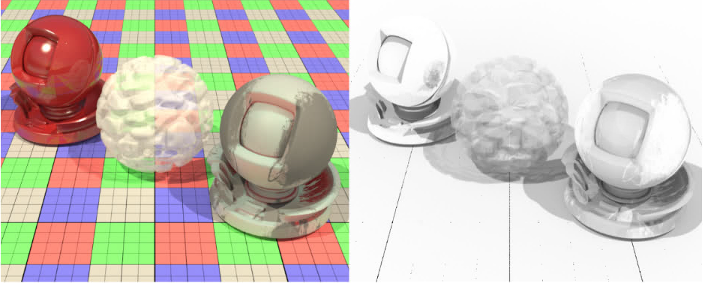# Visibility AOVsA visibility AOV provides a way to visualize how much of the scene is visible from a certain light. In MoonRay, a ratio is calculated representing how much light is received:

``````// # light samples that hit light / total valid light samples
p = hits / total_attempts
// variance of a Bernoulli distribution
result = p * (1 - p)
``````

## Examples

A visibility AOV is composed of a light path expression that defines the set of all paths used to compute the visibility ratio.

``````RectLight("lgt_key") {
["label"] = "lgt_key",
...
}
RenderOutput("vis_lgt_key") {
["result"] = 9, -- visibility aov
["visibility_aov"] = "C[<T.><RS>]*[<R[DG]><TD>]['lgt_key']",
...
}
``````